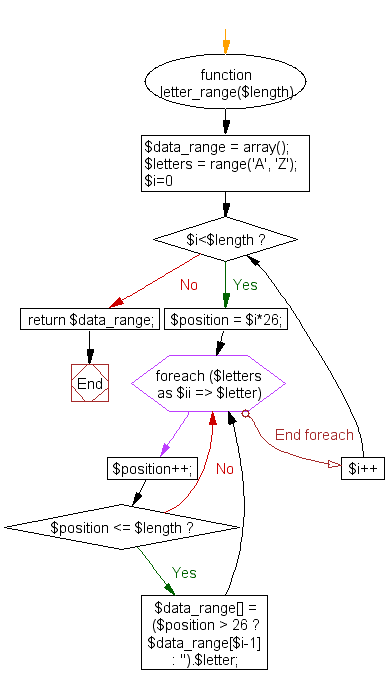﻿ PHP Array Exercise: Create a letter range with arbitrary length - w3resource# PHP Array Exercises : Create a letter range with arbitrary length

## PHP Array: Exercise-30 with Solution

Write a PHP program to create a letter range with arbitrary length.

Sample Solution:

PHP Code:

``````<?php
function letter_range(\$length)
{
\$data_range = array();
\$letters = range('A', 'Z');
for(\$i=0; \$i<\$length; \$i++)
{
\$position = \$i*26;
foreach(\$letters as \$ii => \$letter)
{
\$position++;
if(\$position <= \$length)
\$data_range[] = (\$position > 26 ? \$data_range[\$i-1] : '').\$letter;
}
}
return \$data_range;
}
print_r(letter_range(7));
?>
```
```

Sample Output:

```Array
(
 => A
 => B
 => C
 => D
 => E
 => F
 => G
)
```

Flowchart:PHP Code Editor:

What is the difficulty level of this exercise?

﻿

## PHP: Tips of the Day

PHP: Extract numbers from a string

```\$str = 'In My Cart : 11 12 items';
preg_match_all('!\d+!', \$str, \$matches);
print_r(\$matches);
```